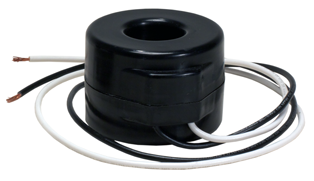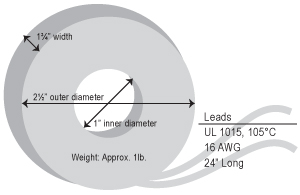# Current Transformers for MeteringThere are two types of electric meters: self-contained (direct drive) and transformer rated.

Most meters used on homes or farms are self-contained. All electric energy used passes through the meter. These meters are designed to be used on services under 200 amperes. Current transformers are contained internally.

When services are more than 200 amperes, transformer-rated meters are used. As the name implies, these types of meters use current transformers (CTs) to measure the current flow, or total power being consumed. The information is recorded by the meter.

In donut-type CTs, there are two conductors, or windings. The primary winding is the line conductor that passes through the center of the CT. The secondary winding is the multiple turns of magnet wire around the core.

The CT transforms the primary current of the line conductor to a smaller, more easily managed current that is carried to the meter which is directly proportional to the primary current. This current is inversely proportional to the number of secondary turns of wire around the iron core.

For a 200:5A CT, the turns ratio is 40:1, giving the secondary current 1/40th of the primary current. For a 400:5A CT, the turns ratio is 80:1, giving a secondary current of 1/80th of the primary current.

The Burden rating (B) is the impedance of the circuit connected to the secondary winding. This impedance is the total opposing effect to the flow of current in an AC circuit. The Burden rating is the maximum amount of impedance before exceeding the minimum accuracy limits.

The current ratio difference between the actual current (primary) and the metered current (secondary) results in what is normally referred to as the multiplier. The correction factor is the factor by which the reading of a watthour meter must be multiplied to correct for the effects of the error ratio and phase angle of the CT.